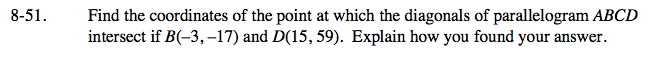Home > GC > Chapter 8 > Lesson 8.1.5 > Problem8-51

8-51.

Find the coordinates of the point at which the diagonals of parallelogram ABCD intersect if B(−3, −17) and D(15, 59). Explain how you found your answer. 8-51 HW eTool (Desmos). Homework Help ✎The diagonals of a parallelogram bisect each other.
That means they have the same midpoint.

See the Math Notes box in Lesson 7.3.3 for help finding the midpoint of the line segment which connects B and D.

The midpoint is (6, 21).

Use the eTool below to explore the diagonals of a parallelogram.
Click the link at right for the full version of the eTool: GC 8-51 HW eTool